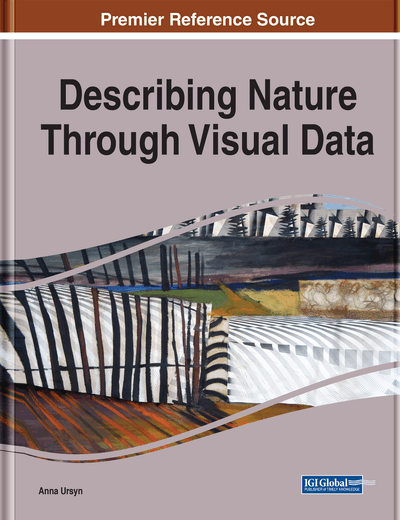# Visualisation of Mathematical Thinking

Hervé Lehning
DOI: 10.4018/978-1-7998-5753-2.ch003
OnDemand:
(Individual Chapters)
Available
\$37.50
No Current Special Offers

## Abstract

Drawing is not proving. For a long time, this argument has been used to avoid the use of visualisation in mathematics. Nevertheless, a number of proofs, concepts, and ideas are easier to understand with the help of a small drawing. In this chapter, the authors show that visualisation in mathematics is helpful not only to illustrate but also to create ideas, and this at all levels.
Chapter Preview
Top

## Introduction

The scene took place at the time of my studies. My professor was at the blackboard, in a packed lecture hall. In front of his fascinated students, he was proving a deep theorem of geometry, using number of diagrams he drew with confidence. The blackboard was becoming white but, suddenly, he stopped in the middle of a diagram (see Figure1).

As time went by, the professor looked more and more puzzled. After a while, he started to make a little drawing, unfortunately hidden by his body. Suddenly, he looked illuminated, erased his drawing and resumed his proof with number of diagrams; we noted them without understanding well. At the end of the lecture, the bravest students went to the desk to ask him some explanations about his little drawing. His reply was unequivocal: “there’s no question to fill your spirit with bad habits of thought”. His reason to refuse was his pedagogical ideas: we must be freed of the errors of the past, and among them of the habit of using drawings to help intuition.

Top

## Proofs Without Words

This conception of mathematics was dominant at the age of what was called “modern mathematics” or “new math” (see ). Nevertheless, number of results has visual proofs (see ). The simplest of them is probably the calculation of the sum of the first natural numbers as: 1 + 2 + 3 + 4 + 5. Of course, in this case, we find 15 easily but it will be more difficult to compute: 1 + 2 + 3 + 4 + … + 100. The general case: 1 + 2 + 3 + 4 + … + n is even more complex. The idea to compute it easily is to model this sum as the area of a staircase (see Figure 2).

By copying the staircase upside-down, we get a rectangle (see Figure 3).

Thus, twice the sum: 1 + 2 + 3 + 4 + 5 + 6 equals the area of the rectangle with side-lengths 6 and 7, which is 42 thus: 1 + 2 + 3 + 4 + 5 + 6 = 21. For the same reason:. Today, this kind of proofs without words is generally accepted when they concern natural numbers (that is to say: 1, 2, 3, etc.). The same technique allows us to prove that the sum of the first n odd numbers equals the square of n (see Figure 4).

The identity: (a + b)2 = a2 + 2 ab + b2 has a proof that, a priori, looks of the same kind. If the sides of the blue and orange squares are a and b, their areas equal a2 and b2 while those of the green rectangles equal the product ab (see Figure5).

## Key Terms in this Chapter

Convergence: The notion of convergence refers to the notion that some sequences or functions approach a certain limit. There are several notions of convergence: pointwise convergence, uniform convergence, etc.

Theorem: A proved mathematical statement. A non-proved mathematical statement thought to be true is called a conjecture.

Dynamical System: A dynamical system is a way of defining a sequence of numbers, points, functions, or curves through a fixed deterministic rule. It is widely used in mathematical modelling.

Computer Algebra System: A piece of software designed to do numerical and formal computations.

Algorithm: The mathematical and rigorous version of a recipe.

Factorisation: A natural number as 1, 2, 3, 4, 5, 6, etc. is a prime number as 2, 3 and 5 with no factor or can be factorized as: 4 = 2 x 2, 6 = 2 x 3 or 153 = 3 x 3 x 17.

Periodic: A sequence or function is periodic if its values repeat at a regular interval (the period).

Anamorphosis: A distorted visualisation.

Proof (Mathematical Proof): A deductive argument for a mathematical statement, starting from axioms (i.e. statements considered as true) and using logic.

## Complete Chapter List

Search this Book:
Reset